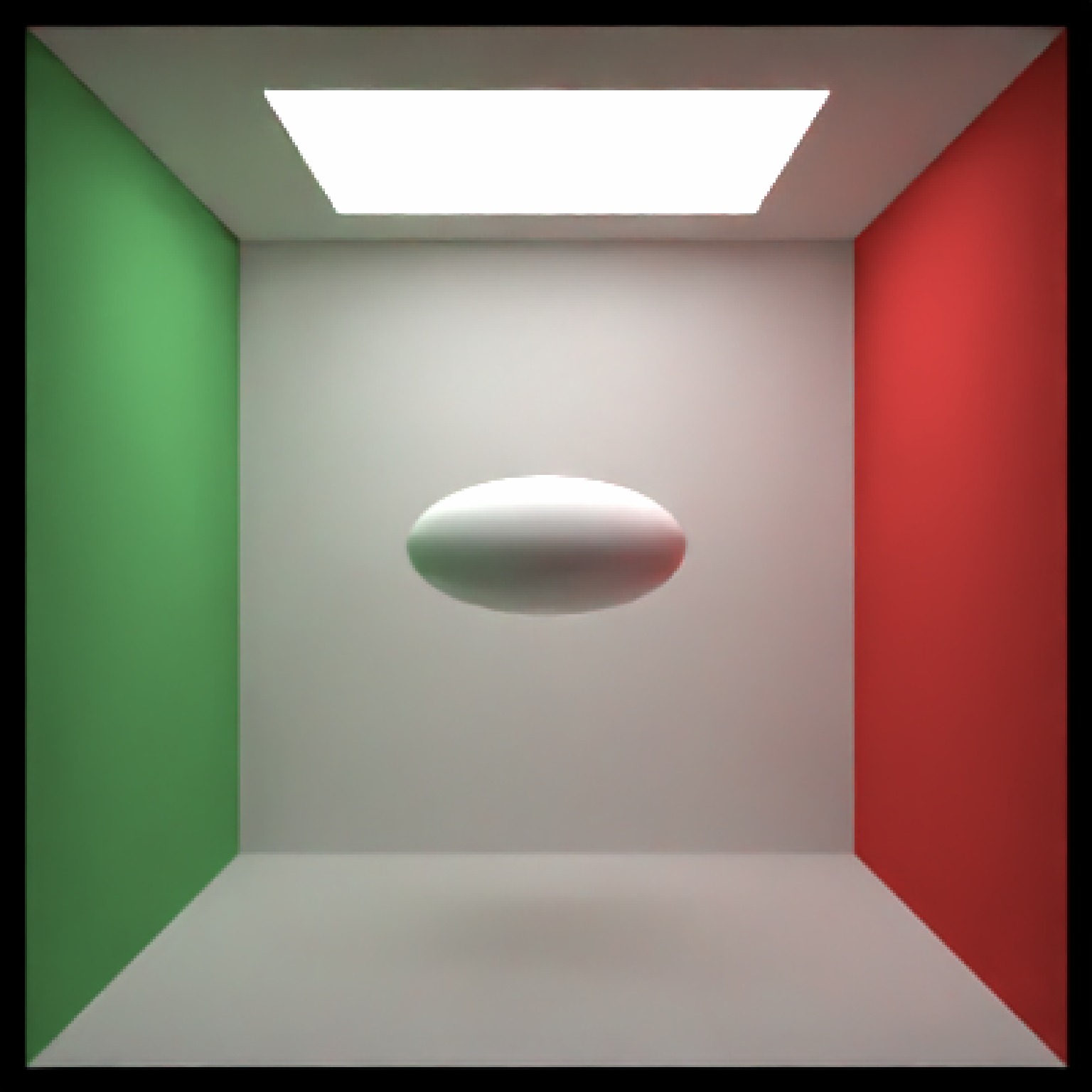Note: light importance sampling for this shape is currently approximated by a sphere. This will fail for ellipsoids with large differences between axes.

ellipsoid(
x = 0,
y = 0,
z = 0,
a = 1,
b = 1,
c = 1,
material = diffuse(),
angle = c(0, 0, 0),
order_rotation = c(1, 2, 3),
flipped = FALSE,
scale = c(1, 1, 1)
)

## Arguments

x

Default 0. x-coordinate of the center of the ellipsoid.

y

Default 0. y-coordinate of the center of the ellipsoid.

z

Default 0. z-coordinate of the center of the ellipsoid.

a

Default 1. Principal x-axis of the ellipsoid.

b

Default 1. Principal y-axis of the ellipsoid.

c

Default 1. Principal z-axis of the ellipsoid.

material

Default diffuse.The material, called from one of the material functions diffuse, metal, or dielectric.

angle

Default c(0, 0, 0). Angle of rotation around the x, y, and z axes, applied in the order specified in order_rotation.

order_rotation

Default c(1, 2, 3). The order to apply the rotations, referring to "x", "y", and "z".

flipped

Default FALSE. Whether to flip the normals.

scale

Default c(1, 1, 1). Scale transformation in the x, y, and z directions. If this is a single value, number, the object will be scaled uniformly. Note: emissive objects may not currently function correctly when scaled.

## Value

Single row of a tibble describing the ellipsoid in the scene.

## Examples

#Generate an ellipsoid in a Cornell box
if(run_documentation()) {
generate_cornell() %>%
add_object(ellipsoid(x = 555/2, y = 555/2, z = 555/2,
a = 100, b = 50, c = 50)) %>%
render_scene(lookfrom = c(278, 278, -800) ,lookat = c(278, 278, 0), fov = 40,
ambient_light = FALSE, samples = 128, parallel = TRUE, clamp_value = 5)
}#Change the axes to make it taller rather than wide:
if(run_documentation()) {
generate_cornell() %>%
add_object(ellipsoid(x = 555/2, y = 555/2, z = 555/2,
a = 100, b = 200, c = 100, material = metal())) %>%
render_scene(lookfrom = c(278, 278, -800) ,lookat = c(278, 278, 0), fov = 40,
ambient_light = FALSE, samples = 128, parallel = TRUE, clamp_value = 5)
}#Rotate it and make it dielectric:
if(run_documentation()) {
generate_cornell() %>%
add_object(ellipsoid(x = 555/2, y = 555/2, z = 555/2,
a = 100, b = 200, c = 100, angle = c(0, 0, 45),
material = dielectric())) %>%
render_scene(lookfrom = c(278, 278, -800) ,lookat = c(278, 278, 0), fov = 40,
ambient_light = FALSE, samples = 128, parallel = TRUE, clamp_value = 5)
}